# Estimating Transfer Function Models for a Boost Converter

This example shows how to estimate a transfer function from frequency response data. You use Simulink® Control Design™ to collect frequency response data from a Simulink model and the `tfest` command to estimate a transfer function from the measured data. To run the example with previously saved frequency response data start from the Estimating a Transfer Function section.

### Boost Converter

```mdl = 'iddemo_boost_converter'; open_system(mdl); ```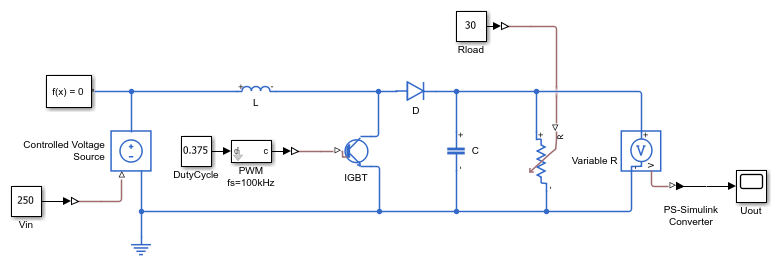The model is of a Boost Converter circuit that converts a DC voltage to another DC voltage (typically a higher voltage) by controlled chopping or switching of the source voltage. In this model an IGBT driven by a PWM signal is used for switching.

For this example we are interested in the transfer function from the PWM duty cycle set-point to the load voltage, Uout.

### Collect Frequency Response Data

We use the `frestimate` command to perturb the duty cycle set-point with sinusoids of different frequencies and log the resulting load voltage. From these we find how the system modifies the magnitude and phase of the injected sinusoids giving us discrete points on the frequency response.

Using `frestimate` requires two preliminary steps

• Specifying the frequency response input and output points

• Defining the sinusoids to inject at the input point

The frequency response input and output points are created using the `linio` command and for this example are the outputs of the `DutyCycle` and `Voltage Measurement` blocks.

```ios = [... linio([mdl,'/DutyCycle'],1,'input'); ... linio([mdl,'/PS-Simulink Converter'],1,'output')]; ```

We use the `frest.Sinestream` command to define the sinusoids to inject at the input point. We are interested in the frequency range 200 to 20k rad/s, and want to perturb the duty cycle by 0.03.

```f = logspace(log10(200),log10(20000),10); in = frest.Sinestream('Frequency',f,'Amplitude',0.03); ```

The simulation time required to simulate the model with this sine-stream signal is determined using the `getSimulationTime` command and as we know the simulation end time used by the model we can get an idea of how much longer the sine-stream simulation will take over simply running the model.

```getSimulationTime(in)/0.02 ```
```ans = 15.5933 ```

We use the defined inputs and sine-stream with `frestimate` to compute discrete points on the frequency response.

```[sysData,simlog] = frestimate(mdl,ios,in); bopt = bodeoptions; bopt.Grid = 'on'; bopt.PhaseMatching = 'on'; figure, bode(sysData,'*r',bopt) ```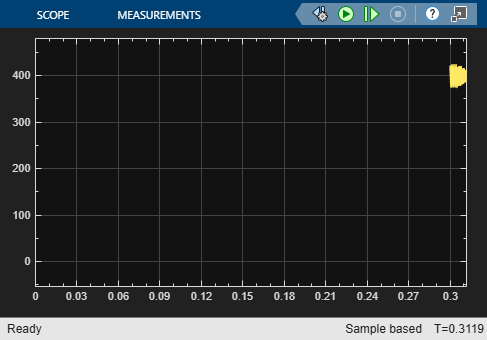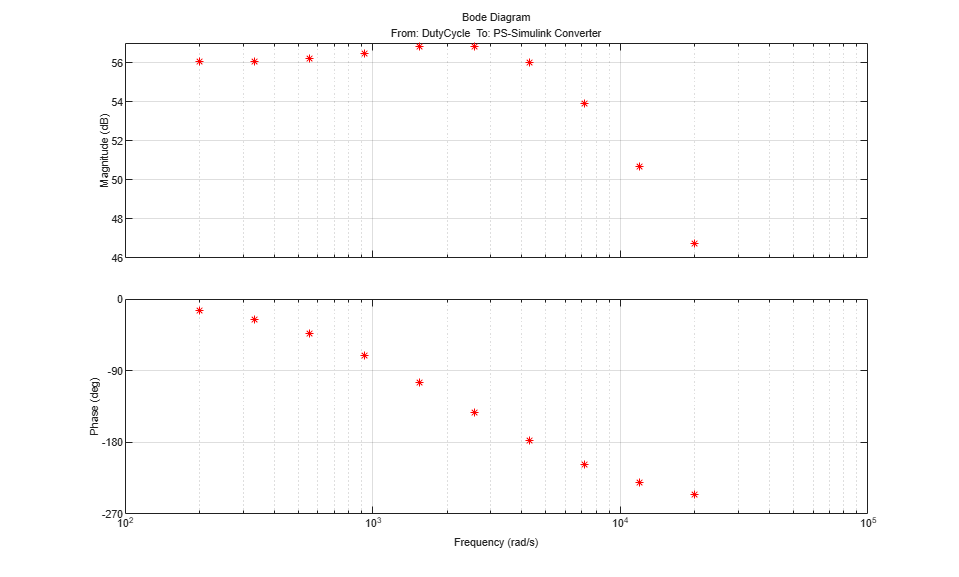The Bode response shows a system with a 56db gain, some minor resonance around 2500 rad/s and a high frequency roll off of around 20 db/decade matching what we would expect for this circuit.

The `frest.simView` command allows us to inspect the frestimate process showing the injected signal, measured output, and frequency response in one graphical interface.

```frest.simView(simlog,in,sysData); ```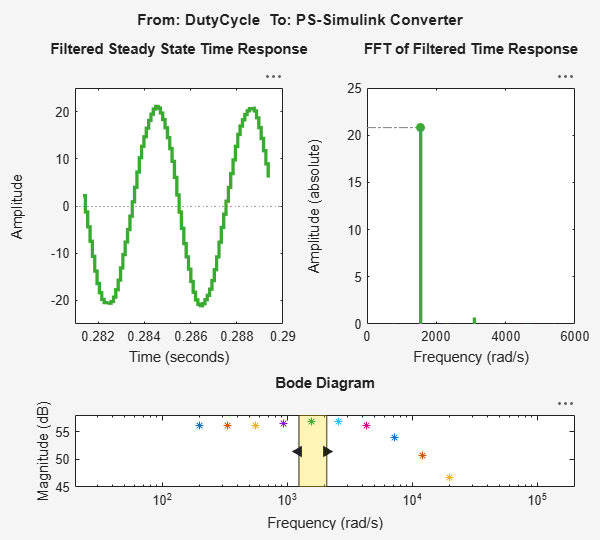The figure shows the model response to injected sinusoids and the FFT of the model response. Notice that the injected sinusoids result in signals with a dominant frequency and limited harmonics indicating a linear model and successful frequency response data collection.

### Estimating a Transfer Function

In the previous step we collected frequency response data. This data describes the system as discrete frequency points and we now fit a transfer function to the data.

We generated the frequency response data using Simulink Control Design, if Simulink Control Design is not installed use the following command to load the saved frequency response data

```load iddemo_boostconverter_data ```

Inspecting the frequency response data we expect that the system can be described by a second order system.

```sysA = tfest(sysData,2) figure, bode(sysData,'r*',sysA,bopt) ```
```sysA = From input "DutyCycle" to output "PS-Simulink Converter": -4.456e06 s + 6.175e09 ----------------------- s^2 + 6995 s + 9.834e06 Continuous-time identified transfer function. Parameterization: Number of poles: 2 Number of zeros: 1 Number of free coefficients: 4 Use "tfdata", "getpvec", "getcov" for parameters and their uncertainties. Status: Estimated using TFEST on frequency response data "sysData". Fit to estimation data: 98.04% FPE: 281.4, MSE: 120.6 ```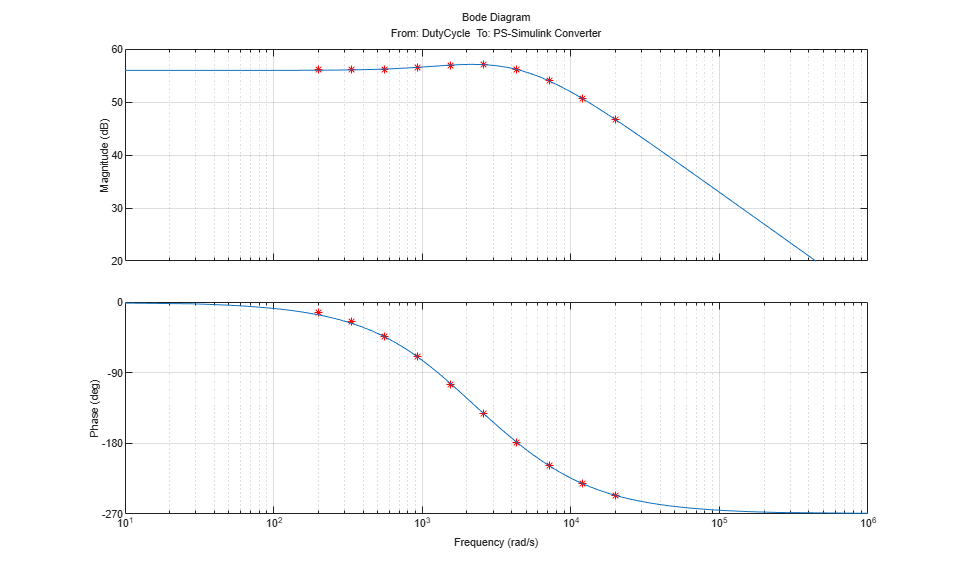The estimated transfer function is accurate over the provided frequency range.

```bdclose(mdl) ```

## SupportGet trial now